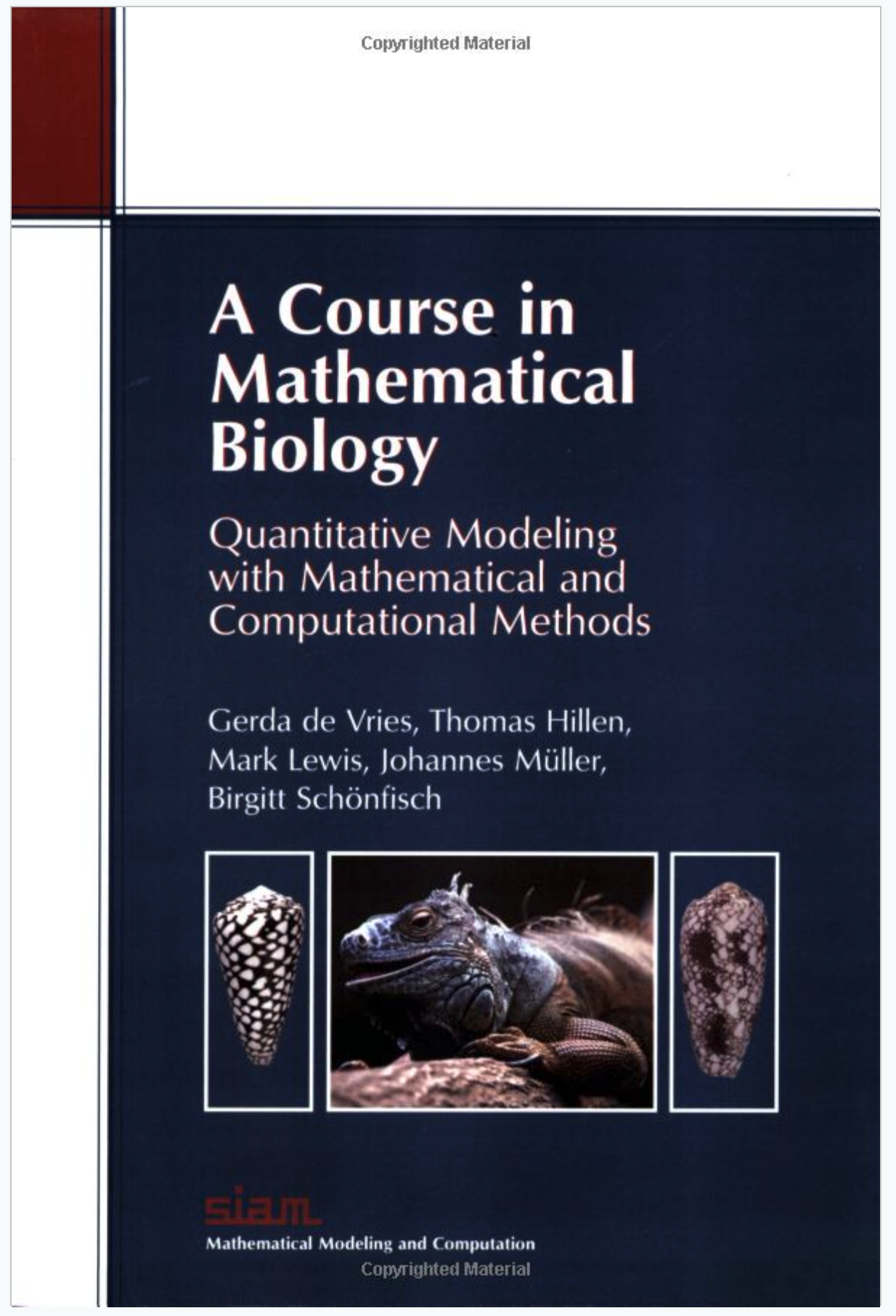G. de Vries

T. Hillen (webpage)

M. Lewis (webpage)

J. Müller (website)

B. Schönfisch

# A Course in Mathematical Biology

#### Quantitative Modeling with Mathematical and Computational Tools

Order through SIAM or Amazon.

Supplemental Material:

 The file  solutions.pdf (the link to the solutions has been temporarily disabled. If you are an instructor and need solutions, please write us an e-mail.)  by A. Beltaos, G de Vries, T. Hillen contains solutions to the following problems: Section 1.4 Modelling Exercise 1 Section 2.4 Difference Equations Exercises: 1, 2, 5, 6, 8, 10, 11, 14, 15, 16 Section 3.9 ODE's Exercises: 1, 2, 3, 4, 5, 6, 9, 13, 17, 18 Section 4.5 PDE's Exercises: 1, 3, 4, 5

 Computer course of Chapter 8 in  MATHEMATICA  by T. de Camino Beck Section8_2.pdf Section8_3.pdf Section8_4.pdf Section8_5.pdf Section8_2.nb Section8_3.nb Section8_4.nb Section8_5.nb

 Computer course of Chapter 8 in  Matlab by T. de Camino Beck and C. Cobbold Matlab_Course.pdf